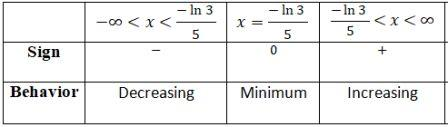# Consider the equation below. f(x) = e^{9x} + e^{-x} (a) Find the interval on which f is...

## Question:

Consider the equation below.

{eq}f(x) = e^{9x} + e^{-x} {/eq}

(a) Find the interval on which {eq}f {/eq} is increasing.

(b) Find the interval on which {eq}f {/eq} is decreasing.

(c) Find the local minimum and maximum values of {eq}f {/eq}.

(d) Find the inflection point.

(e) Find the interval on which {eq}f {/eq} is concave up.

(f) Find the interval on which {eq}f {/eq} is concave down.

## Concavity and Inflection Points

This problem is based on the concept of the concavity and points of inflection. We should be familiar with the concept that at the point of inflection the second derivative of the function is zero. And for maximum or minimum, we can get that from the first derivative test.

The function we have is,

{eq}\displaystyle f(x) = e^{9x} + e^{-x} {/eq}

Part A and Part B: Interval of increase and Decrease

For increase and decrease we need to find the critical point first and for that we need to find the first derivative.

{eq}\displaystyle f'(x) = 9e^{9x} - e^{-x} {/eq}

We can find the critical point as:

{eq}\displaystyle 9e^{9x} - e^{-x} =0 {/eq}

Solving for {eq}\displaystyle x {/eq}, we get,

{eq}\displaystyle x=\frac{-\ln 3}{5} {/eq}

Based on the critical point we can form the intervals of monotone and the table below summarizes the behavior of the function:So,

{eq}\displaystyle \boxed{\text{Intervals of increase}:\left ( \frac{-\ln 3}{5},\infty \right )} {/eq}

{eq}\displaystyle \boxed{\text{Intervals of decrease}:\left ( -\infty,\frac{-\ln 3}{5} \right )} {/eq}

Part C: Local Minimum and Maximum

From the table above we can see that there is no local maximum.

There is a local minimum at {eq}\displaystyle x=\frac{-\ln 3}{5} {/eq}

{eq}\displaystyle \boxed{\displaystyle \text{Minimum}=1.384} {/eq}

Part D: Point of Inflection

Let us calculate the second derivative first because at the point of inflection the second derivative is zero or undefined.

{eq}\displaystyle f''(x)=81e^{9x}+e^{-x} {/eq}

{eq}\displaystyle 81e^{9x}+e^{-x}=0 {/eq}

• No solution
• Thus, no point of inflection for this function.

Part E: Intervals of Concavity

• Since, there is no points of inflection. So,

{eq}\displaystyle \text{Concave up}:(-\infty, \infty) {/eq}

{eq}\displaystyle \text{Concave down}: \text{ nowhere} {/eq}